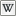# LlEuler2Rot

(diff) ← Older revision | Latest revision (diff) | Newer revision → (diff)

 LSL Portal
Function: rotation llEuler2Rot( vector v );
 16 Function ID 0 Forced Delay 10 Energy

Returns a rotation representation of theEuler Angles v.

 • vector v – Angle

## Specification

The Euler angle vector (in radians) is converted to a rotation by doing the rotations around the 3 axes in Z, Y, X order. So `llEuler2Rot(<1.0, 2.0, 3.0> * DEG_TO_RAD)` generates a rotation by first rotating 3 degrees around the global Z axis, then rotating the result around the global Y axis, and finally rotating that 1 degree around the global X axis.

## Examples

```default
{
state_entry()
{
vector input = <73.0, -63.0, 20.0> * DEG_TO_RAD;
rotation rot = llEuler2Rot(input);
llSay(0,"The Euler2Rot of "+(string)input+" is: "+(string)rot );
}
}
```

```function rotation llEuler2Rot( vector v );